注意

哨兵a0相比insertValue的作用

• 可以省去下边的 j ＞0，因为哨兵就意味着，你走到哨兵那个位置了，就自然要停下来了

力扣912排序数组

/**
* Note: The returned array must be malloced, assume caller calls free().
*/
int* sortArray(int* nums, int numsSize, int* returnSize){
//从第二个数开始遍历
for(int i=1;i<numsSize;i++){
//保存待插入的值
int insertValue=nums[i];
int insertIndex=0;
for(int j=i-1;j>=0;j--){
//若待插入的值小于索引值,证明要索引值需要后移,空出这个位置给插入值
if(insertValue<nums[j]){
nums[j+1]=nums[j];
//记录待插入的位置
insertIndex=j;
}
}
//跳出循环后,把这个数插入到指定位置
nums[insertIndex]=insertValue;
}
/*returnSize = (int*)malloc(numsSize*sizeof(int));
for(int i=0;i<numsSize;i++){
returnSize[i]=nums[i];
}*/
*returnSize=numsSize;
return nums;
}

优化

for循环加了条件,insertValue<nums[j]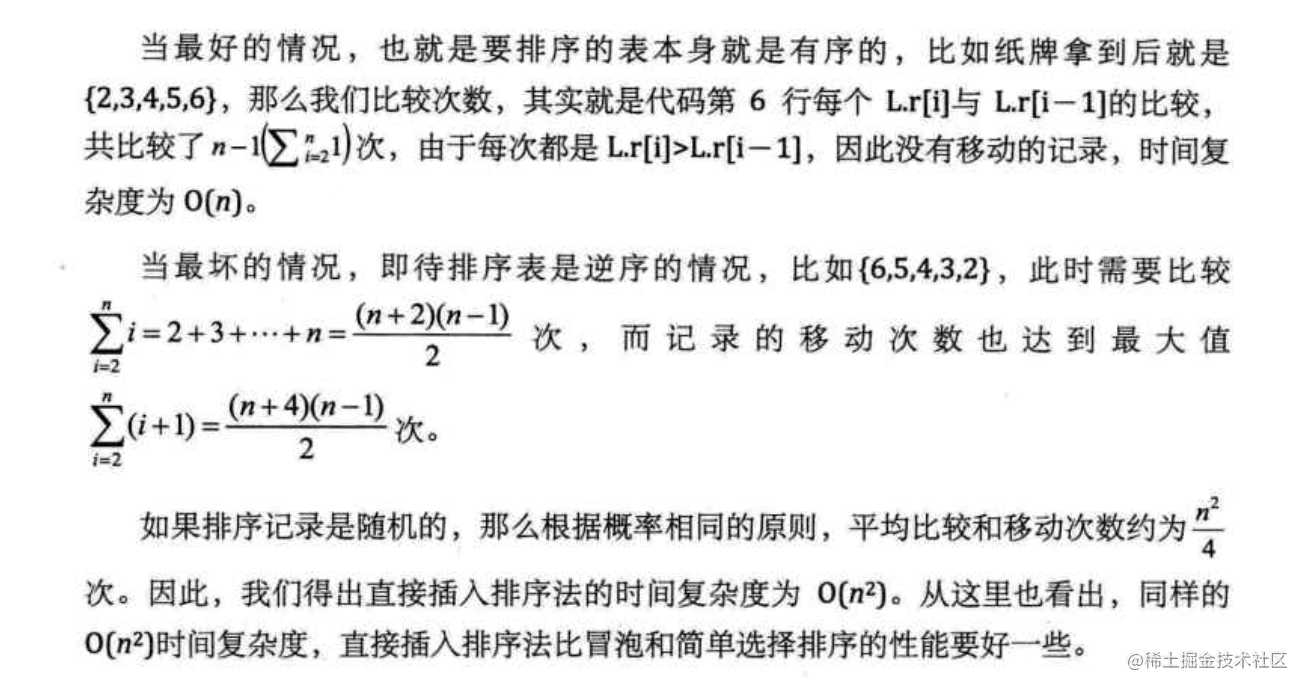空间复杂度:O(1)

/**
* Note: The returned array must be malloced, assume caller calls free().
*/
int* sortArray(int* nums, int numsSize, int* returnSize){
int insertValue = 0;
int j;
//从第二个数开始遍历
for(int i=1;i<numsSize;i++){
//保存待插入的值
insertValue=nums[i];
//因为本身有序,若待插入的数还大于最后一个数,则无须继续遍历下去了
for(j=i-1;j>=0 && insertValue<nums[j];j--){
//若待插入的值小于索引值,证明要索引值需要后移,空出j这个位置给插入值
nums[j+1]=nums[j];
}
//跳出循环后,把这个数插入到指定位置
nums[j+1]=insertValue;
}
/*returnSize = (int*)malloc(numsSize*sizeof(int));
for(int i=0;i<numsSize;i++){
returnSize[i]=nums[i];
}*/
*returnSize=numsSize;
return nums;
}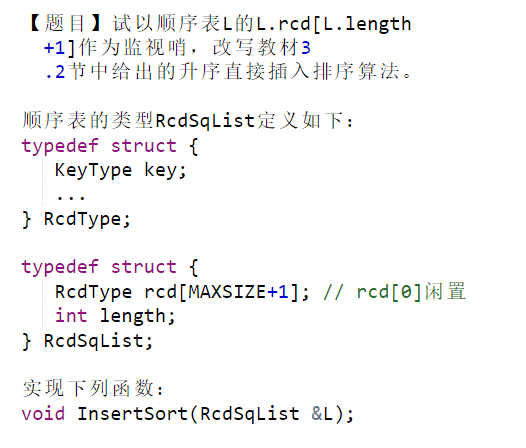哨兵设置在0的方法

#include "allinclude.h"  //DO NOT edit this line
void InsertSort(RcdSqList &L)
{ // Add your code here
for(int i=2;i<=L.length;i++){
int j=i-1;
//记录哨兵
L.rcd.key=L.rcd[i].key;
//找到要插入的位置(往哨兵的方向,哨兵在前边)
while(L.rcd[j].key>L.rcd[L.length+1].key){
L.rcd[j+1].key=L.rcd[j].key;
j--;
}
//出循环时,j+1即是要插入的位置
L.rcd[j+1].key=L.rcd[L.length+1].key;
}
}

哨兵设置在length+1

//刚好跟上边相反,i是从后往前去遍历
for(int i=L.length-1;i>=0;i--){
int j=i+1;
//若本身就小于后边一个了,则无须进行任何移动
if(L.rcd[i].key>L.rcd[j].key){
//记录哨兵
L.rcd[L.length+1].key=L.rcd[i].key;
//找到要插入的位置(j是往后去走,因为哨兵在后边)
while(L.rcd[j].key<L.rcd[L.length+1].key){
L.rcd[j-1].key=L.rcd[j].key;
j++;
}
//出循环时,j-1即是要插入的位置
L.rcd[j-1].key=L.rcd[L.length+1].key;
}
}

总结模板

• for(从第二个开始遍历)

• j=i-1; insertVal = a [i]
• while(j>=0 && insertVal < a[j] )
• a[j+1]=insertVal

力扣147对链表进行插入排序

https://leetcode-cn.com/problems/insertion-sort-list/

超时版本(老老实实从头往前找)

/**
* struct ListNode {
*     int val;
*     struct ListNode *next;
* };
*/

//待插入元素p(直接从第二个元素开始)
while(p){
struct ListNode* pos=NULL;
//若还未遍历到待插入元素的上一个位置
while(q->next!=p){
//若遍历到的当前元素的下一位大于待插入元素,则应将待插入元素插到当前元素的下一位
if(q->next->val > p->val){
//记录待插入位置
pos=q;
//得跳出阿!因为跟之前从后往前找不一样,这里是从前往后找,比如原本是 2 3 4 1
//发现2>1,此时就已经是正确位置了,得跳出
break;
}
//移动q
q=q->next;
}
//再让q移动到p的前一个位置,方便操作
while(q->next!=p){
q=q->next;
}
//出循环时,q已经到待插入元素p的前一位
if(pos!=NULL){
//注意顺序
//先让q的next=p的next
q->next=p->next;
p->next=pos->next;
pos->next=p;
}
//移动p
p=p->next;
}
}

用pre指针,每次遍历的时候记录一下前驱即可

/**
* struct ListNode {
*     int val;
*     struct ListNode *next;
* };
*/

//待插入元素p(直接从第二个元素开始)
//记录待插入元素的前驱,记得初始化为第一个元素!!!
while(p){
//先跟前驱比较
if(p->val>pre->val){
pre=p;
p=p->next;
continue;
}
struct ListNode* pos=NULL;
//若还未遍历到待插入元素的上一个位置
while(q->next!=p){
//若遍历到的当前元素的下一位大于待插入元素,则应将待插入元素插到当前元素的下一位
if(q->next->val > p->val){
//记录待插入位置
pos=q;
//得跳出阿!因为跟之前从后往前找不一样,这里是从前往后找,比如原本是 2 3 4 1
//发现2>1,此时就已经是正确位置了,得跳出
break;
}
//移动q
q=q->next;
}
//让q移动到p的前一个位置(因为前边break了),方便操作
while(q->next!=p){
q=q->next;
}
//出循环时,q已经到待插入元素p的前一位
if(pos!=NULL){
//注意顺序
//先让q的next=p的next
q->next=p->next;
p->next=pos->next;
pos->next=p;
}
//更新前驱
pre=p;
//移动p
p=p->next;
}
}

耗时360ms左右时间优化(判断前驱放在while而不要用continue)

/**
* struct ListNode {
*     int val;
*     struct ListNode *next;
* };
*/

//待插入元素p(直接从第二个元素开始)
//记录待插入元素的前驱,记得初始化为第一个元素!!!
struct ListNode* q;
struct ListNode* pos;
while(p){
// //先跟前驱比较(这样效率低了好多,这样要300ms,换成下边while判断只需要40ms)
// if(p->val>pre->val){
//     pre=p;
//     p=p->next;
//     continue;
// }
pos=NULL;
//先判断是否小于前驱,不是的话直接不用进循环了!!!
//若还未遍历到待插入元素的上一个位置
while(q->next!=p&&p->val<pre->val){
//若遍历到的当前元素的下一位大于待插入元素,则应将待插入元素插到当前元素的下一位
if(q->next->val > p->val){
//记录待插入位置
pos=q;
//得跳出阿!因为跟之前从后往前找不一样,这里是从前往后找,比如原本是 2 3 4 1
//发现2>1,此时就已经是正确位置了,得跳出
break;
}
//移动q
q=q->next;
}
//没必要了,因为有pre可以记录p的上一个位置了
// //让q移动到p的前一个位置(因为前边break了),方便操作
// while(q->next!=p){
//     q=q->next;
// }
//出循环时,判断pos,得知是否需要插入
if(pos!=NULL){
//注意顺序
//先让q的next=p的next
pre->next=p->next;
p->next=pos->next;
pos->next=p;
}
//更新前驱
pre=p;
//移动p
p=p->next;
}
}

耗时40ms左右最外层放个if，时间最优(36ms)

/**
* struct ListNode {
*     int val;
*     struct ListNode *next;
* };
*/

//待插入元素p(直接从第二个元素开始)
//记录待插入元素的前驱,记得初始化为第一个元素!!!
struct ListNode* q;
struct ListNode* pos;
while(p){
// //先跟前驱比较
// if(p->val>pre->val){
//     pre=p;
//     p=p->next;
//     continue;
// }
//直接在这里进行比较,尽量不要continue
if(p->val<pre->val){
pos=NULL;
//若还未遍历到待插入元素的上一个位置
while(q->next!=p){

//若遍历到的当前元素的下一位大于待插入元素,则应将待插入元素插到当前元素的下一位
if(q->next->val > p->val){
//记录待插入位置
pos=q;
//得跳出阿!因为跟之前从后往前找不一样,这里是从前往后找,比如原本是 2 3 4 1
//发现2>1,此时就已经是正确位置了,得跳出
break;
}
//移动q
q=q->next;
}
// //让q移动到p的前一个位置(因为前边break了),方便操作
// while(q->next!=p){
//     q=q->next;
// }
//出循环时,q已经到待插入元素p的前一位
if(pos!=NULL){
//注意顺序
//先让q的next=p的next
pre->next=p->next;
p->next=pos->next;
pos->next=p;
}
}
//更新前驱
pre=p;
//移动p
p=p->next;

}
}Continue耗时测试

测试方法

#include <ctime>
#include <string>
#include <chrono>
#include <sstream>
#include <iomanip>
#include <iostream>

// use strftime to format time_t into a "date time"
std::string date_time(std::time_t posix)
{
char buf; // big enough for 2015-07-08 10:06:51\0
std::tm tp = *std::localtime(&posix);
return {buf, std::strftime(buf, sizeof(buf), "%F %T", &tp)};
}

std::string stamp()
{
using namespace std;
using namespace std::chrono;

// get absolute wall time
auto now = system_clock::now();

// find the number of milliseconds
auto ms = duration_cast<milliseconds>(now.time_since_epoch()) % 1000;

// build output string
std::ostringstream oss;
oss.fill('0');

// convert absolute time to time_t seconds
// and convert to "date time"
oss << date_time(system_clock::to_time_t(now));
oss << '.' << setw(3) << ms.count();

return oss.str();
}

int main()
{
std::cout << stamp() << '\n';
}

用一个有9999个元素的链表测试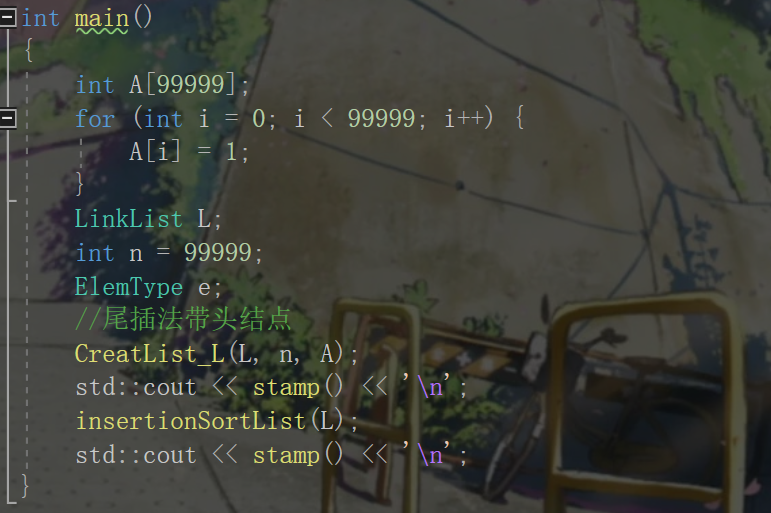Continue版本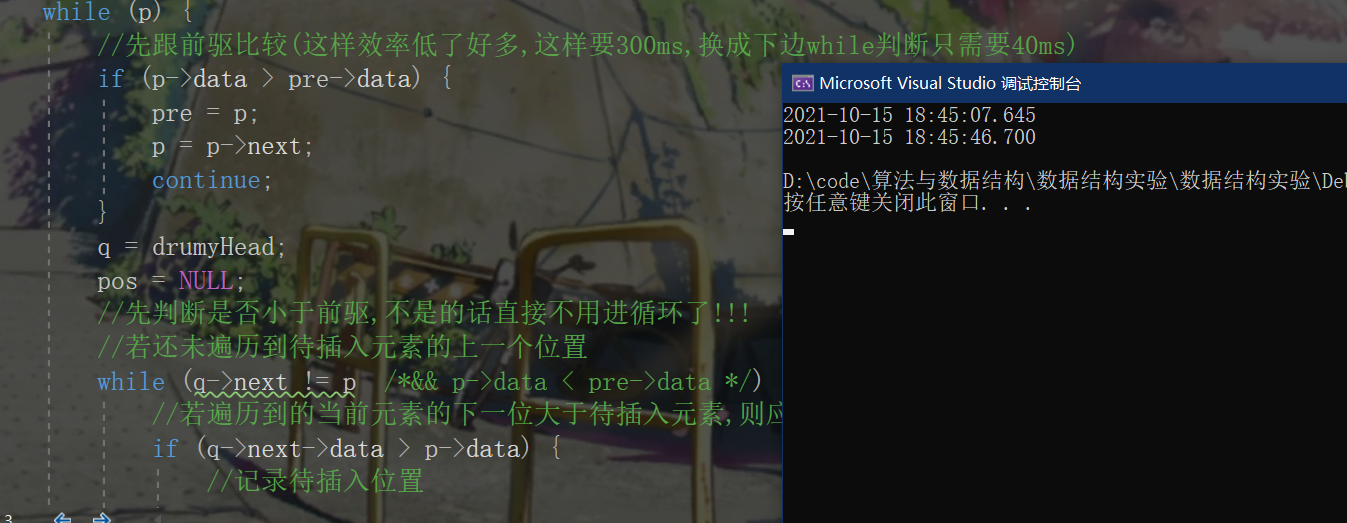上述最优版本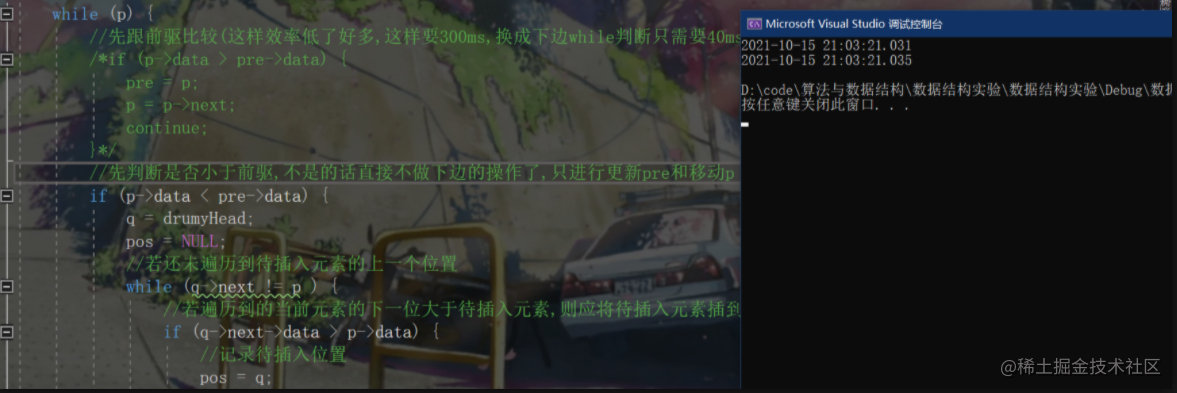结论

• 用continue似乎真的耗时会多很多，不仅在LeetCode上是如此，本机测试也是这般（只不过本机测试可能存在误差），但在网络上还没找到特定的资料佐证这一点的，希望有对这方面了解过的小伙伴可以互相交流探讨一下。

注意

虚拟头的巧用

LeetCode上有时给我们的都是不带头结点的链表,我们可以自己构造一个虚拟头结点!

写在最后

• 关于排序这一章其实还有很多内容,后续也还会更新一下希尔排序,快速排序,归并排序等内容!
最后发现官方题解耗时平均只需要10ms，给跪了直接。。
posted @ 2021-10-21 13:35  Melo~  阅读(15)  评论(0编辑  收藏  举报